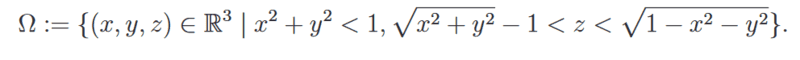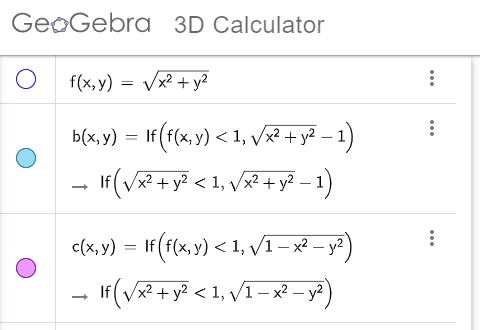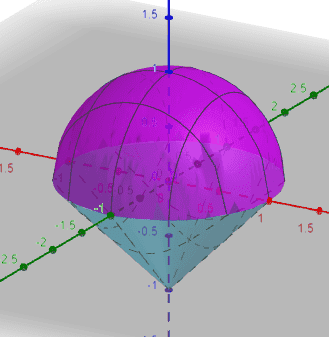# Difficulty visualizing given set

• Physicsdudee
In summary, the conversation discusses the region between a cone and a sphere, bounded by a cylinder and the use of cylindrical coordinates for integration. The participants also mention useful tools such as Wolfram Alpha and GeoGebra for visualizing the set. The conversation ends with a warm welcome to a new member of the Physics Forums community.

#### Physicsdudee

Homework Statement
Hey,
As you can see in the attachment, I am given a Set that I should integrate a function on. The integrating part should not be a problem, however I am having a hard time figuring out how this set looks like and hence which coordinates to use. I get that the best option would probably be spherical coordinates, but I have to have an idea how this set looks like so I can determine the bounds of integration, that is bounds for φ, Θ and R.
Relevant Equations
Spherical coordinates: x=Rsin(Θ)cos(φ), y=Rsin(Θ)sin(φ), z=Rcos(Θ)
Okay so I know, that if the radius is 0, the z coordinate will run from -1 to +1. If the radius tends to one, the z coordinate will tend to 0.
But I still cannot imagine how this set looks like, help would be appreciated.

Thank you.This is the region between the cone $x^2 + y^2 = (z + 1)^2$ and the sphere $x^2 + y^2 + z^2 = 1$ and bounded by the cylinder $x^2 + y^2 = 1$.

I would suggest cylindrical polars.

•Delta2
If I were to use cylindrical coordinates, then I assume I would let φ range from 0 to 2π, z from -1 to 1. But what about R then? R should be a function of the height regarding integration over the cone and then another function of height regarding integration over the sphere right?

Physicsdudee said:
If I were to use cylindrical coordinates, then I assume I would let φ range from 0 to 2π, z from -1 to 1. But what about R then? R should be a function of the height regarding integration over the cone and then another function of height regarding integration over the sphere right?

Easier to have $0 \leq r \leq 1$, and the $z$ limits are then conveniently set out in the question...

Yes, that seems easier, thanks a lot!

While it's good to try to reason on your own,
be aware that there are useful tools, as @fresh_42 suggests.

Here's another one

https://www.geogebra.org/3d?lang=enI'm sure it can also handle spherical and cylindrical coordinates.

•Delta2
•robphy
robphy said:
While it's good to try to reason on your own,
be aware that there are useful tools, as @fresh_42 suggests.

Here's another one

https://www.geogebra.org/3d?lang=en
View attachment 302958
View attachment 302960

I'm sure it can also handle spherical and cylindrical coordinates.
Yep, I know Geo gebra but I was so eager to actually not use anything because in the exam it’s not going to be any different. But I get your point, making use of all these nice tools is extremely good for visualizing

@Physicsdudee ,
I see that this is the second Thread you have started, but you have not received a formal welcome to Physics Forums. Let's fix that.•Delta2
SammyS said:
@Physicsdudee ,
I see that this is the second Thread you have started, but you have not received a formal welcome to Physics Forums. Let's fix that.Haha that’s so kind, I appreciate it:)

•Delta2